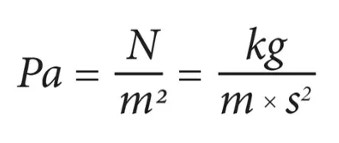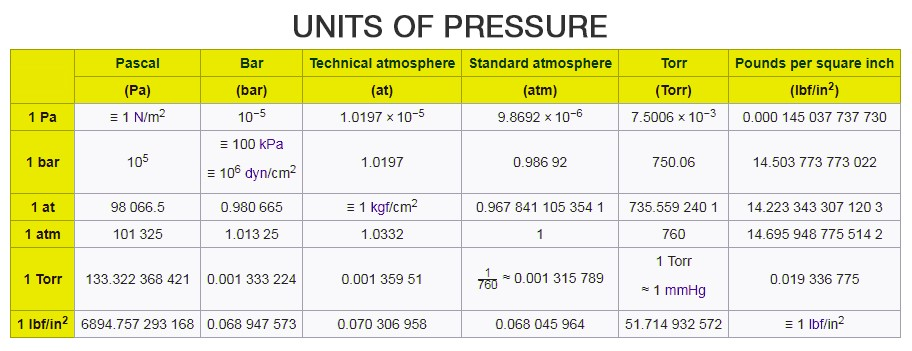# Unit Of Pressure

## Introduction:

Pressure is usually defined as the amount of force applied to a certain area. The pressure is a physical quantity mainly expressed as the formula p = F/A, where F and A are force per area perpendicular to the surface. While we have already discussed everything about pressure in our earlier article, we will explore the topic of units of pressure.

We will look at what is the unit of pressure by first learning about the SI system, which is the International System of Units, derived from the metric system.

• The SI unit also elaborately known as International System of Units has been mainly adopted from French Système international (d’unités).
• This is a modern version of the metric system and is popularly used when measuring physical quantities.
• This system is basically built upon seven base units which are, Metre, Kilogram, Ampere, Kelvin, Second, Candela and Mole.

## What is the SI Unit of Pressure?

The SI unit of pressure is Pascal (represented as Pa) which is equal to one newton per square metre (N/m2 or kg m-1s-2). Interestingly, this name was given in 1971. Before that pressure in SI was measured in newtons per square metre.

### Pascal Formula:### What is the CGS Unit Of Pressure?

The CGS unit of pressure is barye (Ba), equal to 1 dyn·cm−2, or 0.1 Pa.

You may also want to check out these topics given below!

## Other Units Of Pressure

There are several other units of pressure such as pounds per square inch and bar, unit of atmospheric pressure is atm, centimetres of water, millimetres of mercury or inches of mercury (used as a unit of blood pressure), torr, MSW and FSW.## Watch the video and understand fluid pressure.Test your Knowledge on unit of pressure